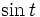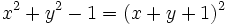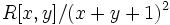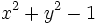# Ring of trigonometric polynomials

This is a variation of polynomial ring
View a complete list of variations of polynomial ring OR read a survey article on varying polynomial ring

## Definition

Let$R$ be a commutative unital ring. The ring of trigonometric polynomials or ring of circular polynomials over$R$ is defined as the ring$R[x,y]/(x^2 + y^2 - 1)$. Equivalently, it is the ring$R[\cos t, \sin t]$ where$\cos t$ and$\sin t$ are subject to the usual relation$\cos^2 t + \sin^2 t = 1$.

## Ring properties

### Affine ring

The ring of trigonometric polynomials is an affine ring over$R$. In particular, when$R$ is a field, the ring of trigonometric polynomials is an affine ring over a field.

Thus, the ring$R[x,y]/(x^2 + y^2 - 1)$ is a Noetherian ring whenever$R$ is a Noetherian ring.

### Integral domain

When$R$ has characteristic two, we have$x^2 + y^2 - 1 = (x + y + 1)^2$, so the quotient is the ring$R[x,y]/(x+y+1)^2$ which is a local ring with unique maximal ideal generated by$x + y + 1$.

On the other hand, when$R$ is a field of characteristic not equal to two, the polynomial$x^2 + y^2 -1$ is irreducible over$R[x,y]$. Thus, the quotient$R[x,y]/(x^2 + y^2 - 1)$. For full proof, refer: Ring of trigonometric polynomials over field of characteristic not equal to two is integral domain

### Unique factorization domain

For$R$ a field of characteristic not equal to two, the ring of trigonometric polynomials over$R$ is a unique factorization domain if and only if$-1$ is a square in the field.